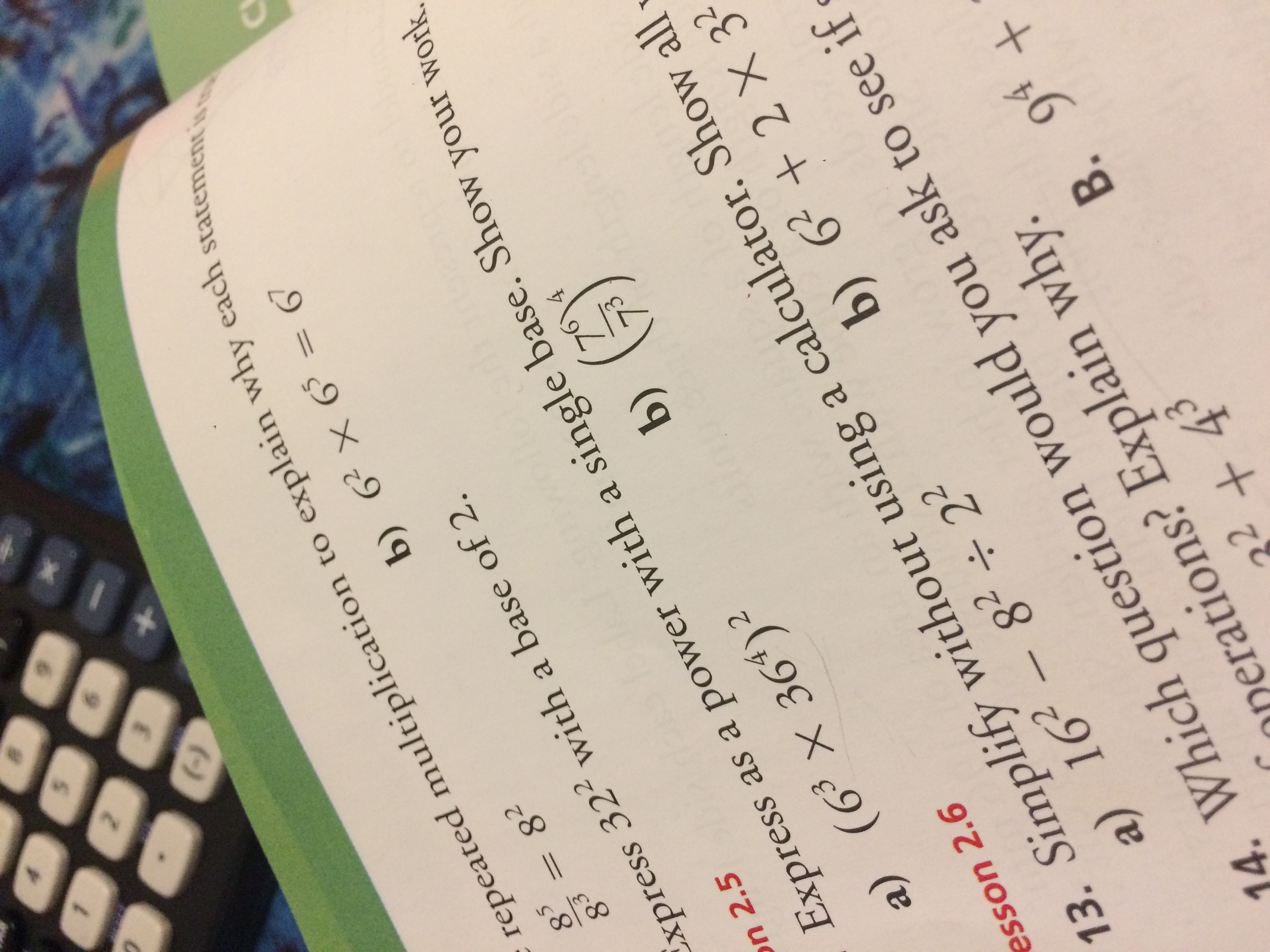# 5N+repeated multiplication to explain why each statement isb) 6 x 658283xpress 32 with a base of 2n 2.5Express as a power with a single base. Show your work.b)4a) (63 x 364)213. Simplify without using a calculator. Show alla) 162 - 8222esson 2.6b) 622 X 32Which question would you ask to see ifperations? Explain why.+434.B. 94

Question
4 views

help on 12 ahelp_outlineImage Transcriptionclose5 N + repeated multiplication to explain why each statement is b) 6 x 65 82 83 xpress 32 with a base of 2 n 2.5 Express as a power with a single base. Show your work. b) 4 a) (63 x 364)2 13. Simplify without using a calculator. Show all a) 162 - 8222 esson 2.6 b) 622 X 32 Which question would you ask to see if perations? Explain why. +43 4. B. 94 fullscreen
check_circle

Step 1

Firstly, we express 36 as 6^2 and use the result that (x^a)^b=x^(ab)

Step 2

Now we use the result ( x^m)*(x^n)=x^(m+n) an...

### Want to see the full answer?

See Solution

#### Want to see this answer and more?

Solutions are written by subject experts who are available 24/7. Questions are typically answered within 1 hour.*

See Solution
*Response times may vary by subject and question.
Tagged in

### Algebra